Open in App
Not now

# How to use Binomial Distribution in Excel?

• Last Updated : 22 Aug, 2022

The BINOM.DIST Function is ordered under Excel Statistical capabilities. It works out the binomial distribution likelihood for the number of triumphs from a predetermined number of preliminaries. This binomial distribution Excel guide will tell you the best way to utilize the capability, bit by bit. The binomial distribution is a factual measure that is regularly used to show the likelihood of a particular number of victories happening from a particular number of free preliminaries. The two structures utilized are:

• The Probability Mass Function: Ascertains the likelihood of there being precisely x triumphs from n autonomous preliminaries.
• The Cumulative Distribution Function: Computes the likelihood of there being all things considered x triumphs from n autonomous preliminaries.

In the monetary examination, the BINOM.DIST capability can help in finding out, for instance, the likelihood of distributing a smash hit book from a scope of books to be distributed by an organization.

## Formula for Binomial Distribution

=BINOM.DIST(number_s,trials,probability_s,cumulative)

The BINOM.DIST utilizes the accompanying contentions:

1. Number_s (required argument): This is the number of accomplishments in preliminaries.
2. Trials (required argument): This is the number of autonomous preliminaries. It should be more noteworthy than or equivalent to 0.
3. Probability_s (required argument): This is the likelihood of progress in every preliminary.
4. Cumulative (required argument): This is a legitimate worth that decides the type of capability. It can either be:
• TRUE: Utilizes the aggregate conveyance capability.
• FALSE: Utilizes the likelihood mass capability.

## How to Use the Binomial Distribution Function in Excel?

To comprehend the purposes of the BINOM.DIST capability, let us think about a model. Example: Assume we are given the accompanying information,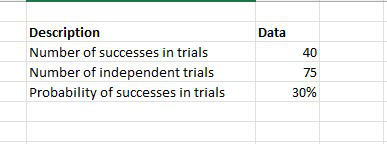Step 1: The equation for ascertaining binomial distribution utilizing the total distribution capability is displayed underneath,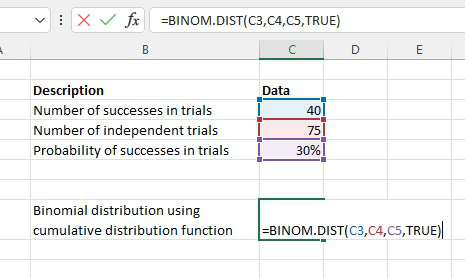Step 2: We come by the outcome underneath.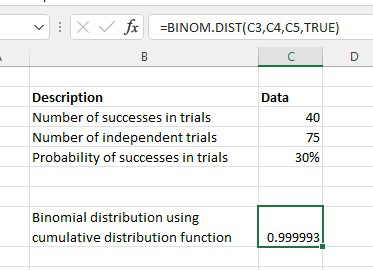Step 3: The recipe for working out binomial distribution utilizing the likelihood mass capability is displayed beneath: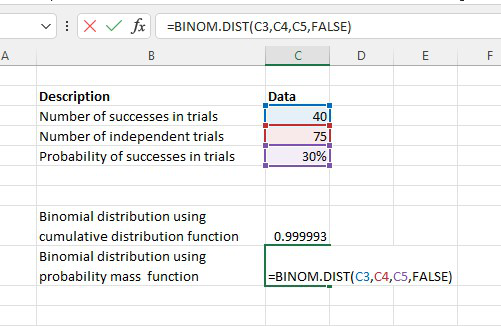Step 4: We come by the outcome beneath.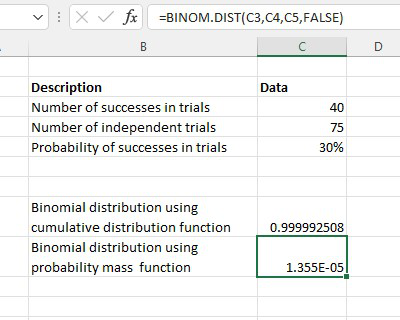### A Few Notes About the BINOM.DIST Function

• The BINOM.DIST capability will shorten all mathematical qualities to Integer.
• #VALUE! error – Occurs when any of the contentions given is non-numeric.
• #NUM! error – Occurs when:
1. The given likelihood is under nothing or more noteworthy than 1.
2. The given number_s is under nothing or more noteworthy than the number of preliminaries.
My Personal Notes arrow_drop_up
Related Articles